Support the Monkey! Tell All your Friends and TeachersHome MonkeyNotes Printable Notes Digital Library Study Guides Study Smart Parents Tips College Planning Test Prep Fun Zone Help / FAQ How to Cite New Title Request

 CHAPTER 9 : APPLICATIONS 9.1 The Expression A sin wt + B cos wt The equation y = A sin wt + B cos wt .........(1) Put A = a cos a and B = a sin a in equation (1) we get y = ( a cos a ) sin wt + (a sin a) cos wt y = a [ sin wt cos a + cos wt sin a] y = a sin (wt + a) ........(2) The two equations (1) and (2) are equivalent expressions.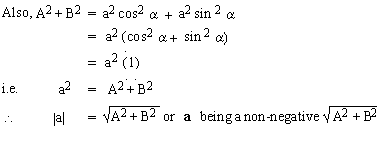and Asin wt + Bcos wt = a sin (wt + a) given that 'a' is an angle with a point P (A, B) on its terminal side.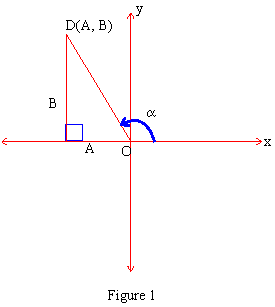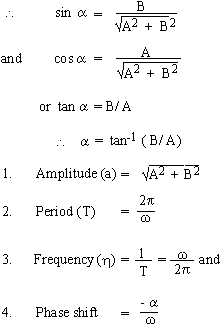Example 1 Transform y = 3 sin 2t + 4 cos 2t to the form y = a sin (wt + a). Also find period, amplitude, frequency and phase shift. Solution y = 3 sin 2t + 4 cos 2t. Comparing with y = A sin wt + B cos wt, we get A = 3, B = 4 and w = 2.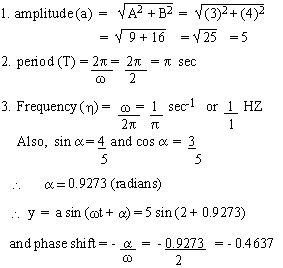Example 2 Convert y = sin p t + cos p t to the form y = a sin (wt + a). Also find its period, frequency, amplitude and phase shift. Solution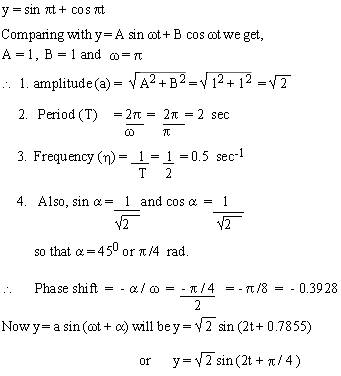Index Chapter 1
 Search: All Products Books Popular Music Classical Music Video DVD Toys & Games Electronics Software Tools & Hardware Outdoor Living Kitchen & Housewares Camera & Photo Cell Phones Keywords: